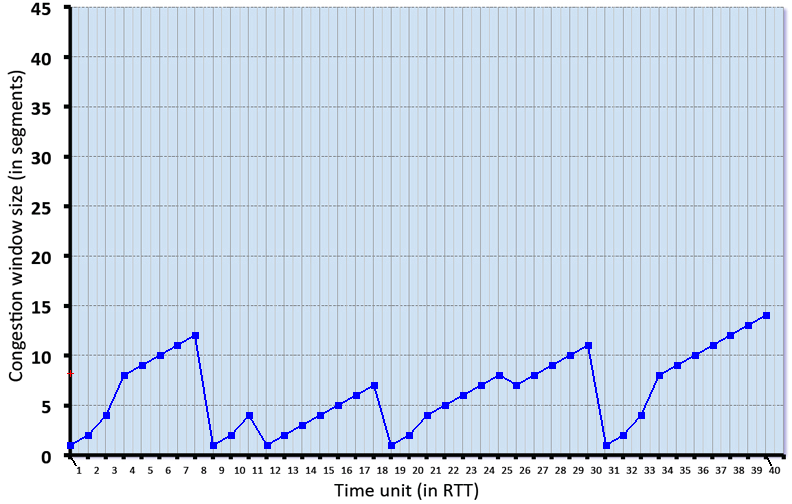# Interactive end-of-chapter exercises

### TCP in Action: slow start, congestion avoidance, and fast retransmit

Consider the figure below, which plots the evolution of TCP's congestion window at the beginning of each time unit (where the unit of time is equal to the RTT); see Figure 3.53 in the text. In the abstract model for this problem, TCP sends a "flight" of packets of size cwnd at the beginning of each time unit. The result of sending that flight of packets is that either (i) all packets are ACKed at the end of the time unit, (ii) there is a timeout for the first packet, or (iii) there is a triple duplicate ACK for the first packet. In this problem, you are asked to reconstruct the sequence of events (ACKs, losses) that resulted in the evolution of TCP's cwnd shown below.Consider the evolution of TCP's congestion window in the example above and answer the following questions. The initial value of cwnd is 1 and the initial value of ssthresh (shown as a red +) is 8.

## Question 1 of 6

We gratefully acknowledge the programming and problem design work of John Broderick (UMass '21), which has really helped to substantially improve this site.